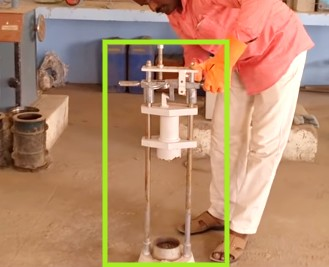# Aggregate impact value test- step by Step Procedure with Report

Table of Contents

## Why do we use aggregate impact value test?

The aggregate impact value test is done to test the compressive strength of aggregate when sudden and immediate impact force acted on it. It is expressed in percentage (%). It should be less for high impact bearing aggregate for pavement.

In this post I will show you the details of the aggregate impact value test. So, read it carefully to get full knowledge.

## Apparatus required

• Aggregate impact value machine whose weight should be between 45 kg to 60 kg.
• A cylindrical steel cup measuring the diameter of 75 mm and depth should be 50 mm.
• Another steel cup whose diameter should be 75 millimeters and depth should be 50 mm.
• The fall of the machine load on the aggregate cup should be 385 millimeters.
• Seive of 12.5 millimeters.
• Second sieve of 10 millimeters.
• Third sieve of 2.36 millimeters.
• 6 digital measuring beam balance.
• Sample container.
• A tamping rod of 10-millimeter diameter and 230-millimeter long.
• Bowl

## Procedure for aggregate impact value testAggregate impact value test Machine

Take a sample of aggregate and sieve with the help of a 12.5-millimeter sieve and retain it into a 10-millimeter sieve.

Sieving should be done in a circular motion.

After that sample should be sieved with 10 millimeters sieve and collect in a container.

Now, the collected sample of aggregate is heated in an oven at 110 degree Celsius till 4 hours.

Now, take out the aggregate sample from the oven and cool it at room temperature.

Take the weight of the cylindrical cup (M).

Now, fill the cup with an aggregate sample in three-layer.

Compact the aggregate in each layer with the help of a tamping rod at least 25 tamp.

Now, finally, remove the upper portion of aggregate and makes the surface of the steel cup plain.

Again, take the weight of the cup with filled aggregate, and weight should be noted (m).

Calculate the weight of the sample (A) = (L-m)

Now, transfer the aggregate sample to the aggregate impact machine cup.

Tamp again at least 25 times with the help of a tamping rod.

Lock the cup in the tamping machine with the help of a screw.

Now, provide 15 blows to the aggregate with the help of the impact machine.

Keep in mind the time difference between two consecutive blows should not be less than 1 second.

Now take out the sample from the impact machine.

Take 2.36-millimeter sieve and sieve the impact aggregate through it.

Now, take a bowl and measure its weight (X).

Put the passed dust of aggregate through 2.36 sieve into a bowl.

Take the weight of the bowl again with the aggregate sample dust (Y).

Calculate the weight of passed dust, B = (X-Y)

Now, the final report of aggregate impact value is calculated as,

## Final report of aggregate impact value test

Weight of oven dried aggregate sample (A) = 332.8 gm.

Weight of fraction (dust) of aggregate passing through 2.36 mm sieve (B) = 23.2 gm

Aggregate impact value,

=(B/A)*100%

=( 23.2/332.8) * 100%

= 6.97%

And, hence, the finally aggregate impact value is 6.97 .

## Significance of impact test

• The procedure of determining aggregate impact value is according to IS 2386.
• More will the impact value more will be the strength of aggregate.
• The elongated and flaky aggregate has low strength as it has a higher surface area and gets more stress on it.
• Different impact value of aggregate should be used for different types of pavement given below.

## Precaution for aggregate impact value test

• The sample of aggregate should be sieved in circular motion or movement.
• The sample aggregate should not be overheated on the oven.
• The weight of samples and dust should be tamped with the help of a standard size tamping rod.
• The hole of sieve should not be torn and should be uniformly bound.
• The bowl for pouring aggregate should be dried initially before pouring sample dust.

Read Also,

Ductility test of bitumen- Step by Step Full Procedure with Apparatus

Penetration test of bitumen- Step by Step Procedure and significance

Softening point test of bitumen- Step by Step Procedure and Significance

10 Cause of crack in concrete structure like Column, Beam, Slab, etc

error: Content is protected !!